``````library(ggcyto)
data(GvHD)
fs <- GvHD[subset(pData(GvHD), Patient %in%5:7 & Visit %in% c(5:6))[["name"]]]
fr <- fs[]``````

### 1d histogram/densityplot

`ggcyto` wrapper will construct the `ggcyto` object that inherits from `ggplot` class.

``````p <- ggcyto(fs, aes(x = `FSC-H`))
class(p)``````
``````##  "ggcyto_flowSet"
## attr(,"package")
##  "ggcyto"``````
``is(p, "ggplot")``
``##  TRUE``

Since only one dimension is specified, we can add any 1d geom layer

``````p1 <- p + geom_histogram()
p1``````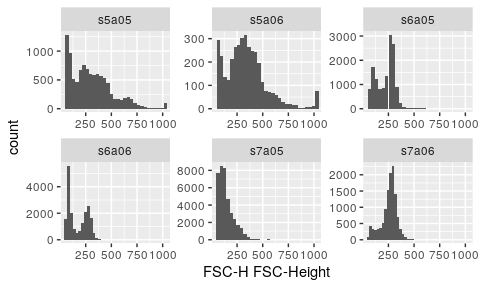As shown, data is facetted by samples name automatically (i.e `facet_wrap(~name)`).

We can overwrite the default faceting by any variables that are defined in `pData`

``pData(fs)``
``````##       Patient Visit Days Grade  name
## s5a05       5     5   19     3 s5a05
## s5a06       5     6   26     3 s5a06
## s6a05       6     5   19     3 s6a05
## s6a06       6     6   27     3 s6a06
## s7a05       7     5   21     3 s7a05
## s7a06       7     6   28     3 s7a06``````
``p1 + facet_grid(Patient~Visit)``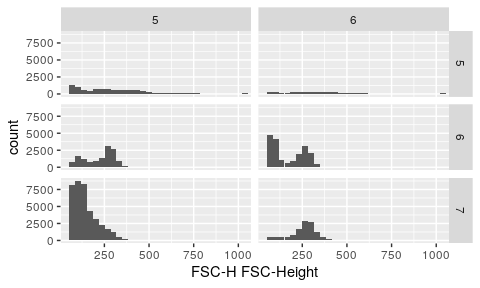To display 1d density

``p + geom_density()``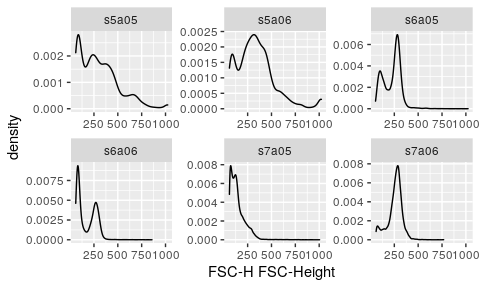Fill the same color

``p + geom_density(fill = "black")``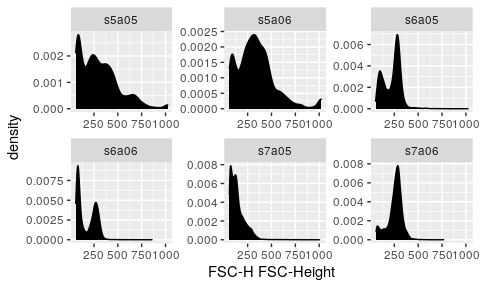Fill different colors

``ggcyto(fs, aes(x = `FSC-H`, fill = name)) + geom_density(alpha = 0.2)``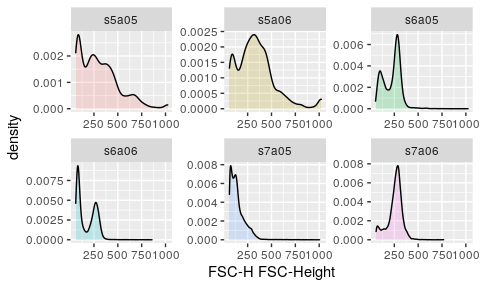Or plot in the same panel by using `ggplot` directly (thus removing the default facetting effect)

``ggplot(fs, aes(x = `FSC-H`, fill = name)) + geom_density(alpha = 0.2)``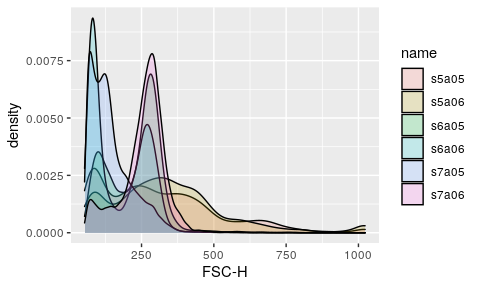### stacked density plot

``````#you can use ggridges package to display stacked density plot
require(ggridges)
#stack by fcs file ('name')
p + geom_density_ridges(aes(y = name)) + facet_null() #facet_null is used to remove the default facet_wrap (by 'name' column)``````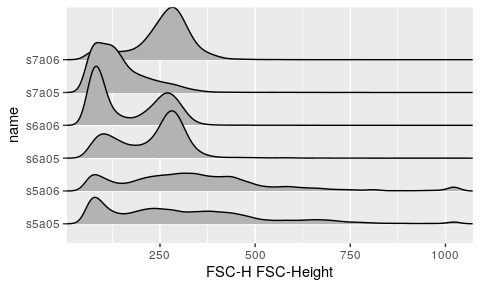``````#or to stack by Visit and facet by patient
p + geom_density_ridges(aes(y = Visit)) + facet_grid(~Patient)``````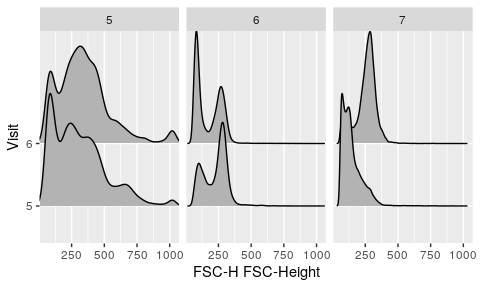### 2d scatter/dot plot

``````# 2d hex
p <- ggcyto(fs, aes(x = `FSC-H`, y =  `SSC-H`))
p <- p + geom_hex(bins = 128)
p``````